## Circuit Analysis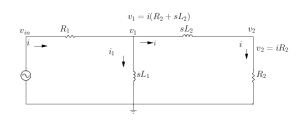Assume a current ‘i’ flows in a particular direction.

The voltages at the nodes have been named as vin, v1,v2

At node v2 :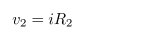At node v1 :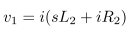Through SL1 :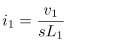At vin :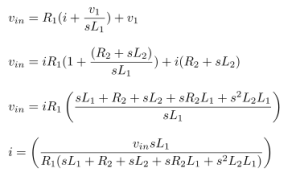v1 and v2 in terms of vin :## Powering up an LED

Powering up an LED

ledcalc.com ; A useful tool to determine the value of resistors to be used in the circuit.

V = I × R (Ohm’s law)

supply voltage = 5V

resistor used = 2 x 33ohm resistor connected in parallel = 16.5 ohm

to determine current ; I = V / R

5 / 16.5 = 300mA

(Power supply voltage − LED voltage) / current (in amps) = desired resistor value (in ohms)

To calculate the amount of power that the resistor will dissipate;

Power Rule: P = (I × V ) W
If a current I flows through through a given element in your circuit, losing voltage V in the process, then the power dissipated by that circuit element is the product of that current and voltage: P = I × V.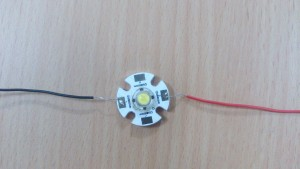Capacitor pdf

# Capacitor

Information

A capacitor (originally known as a condenser) is a passive two-terminal electrical component used to store energy electro statically in an electric field. The forms of practical capacitors vary widely, but all contain at least two electrical conductors (plates) separated by a dielectric (i.e. insulator). The conductors can be thin films, foils or sintered beads of metal or conductive electrolyte, etc. The “non-conducting” dielectric acts to increase the capacitor’s charge capacity. A dielectric can be glass, ceramic, plastic film, air, vacuum, paper, mica, oxide layer etc. Capacitors are widely used as parts of electrical circuits in many common electrical devices. Unlike a resistor, an ideal capacitor does not dissipate energy. Instead, a capacitor stores energy in the form of an electrostatic field between its plates.

When there is a potential difference across the conductors (e.g., when a capacitor is attached across a battery), an electric field develops across the dielectric, causing positive charge +Q to collect on one plate and negative charge −Q to collect on the other plate. If a battery has been attached to a capacitor for a sufficient amount of time, no current can flow through the capacitor. However, if a time-varying voltage is applied across the leads of the capacitor, a displacement current can flow.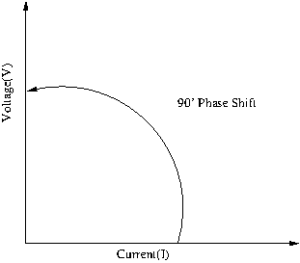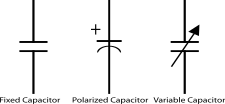Capacitors are widely used in electronic circuits for blocking direct current while allowing alternating current to pass. In analogue filter networks, they smooth the output of power supplies. In resonant circuits they tune radios to particular frequencies. In electric power transmission systems, they stabilize voltage and power flow.

An ideal capacitor is wholly characterized by a constant capacitance C, defined as the ratio of charge ±Q on each conductor to the voltage V between them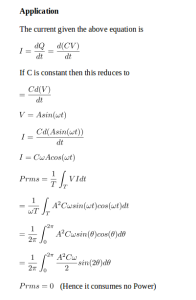## Nullator and Norator

Nullator

In electronics, a nullator is a theoretical linear, time-invariant one port defined as having zero current and voltage across its terminals. Nullators are strange in the sense that they simultaneously have properties of both a short (zero voltage) and an open current(zero current). They are neither current nor voltage sources, yet both at the same time.

Inserting a nullator in a circuit schematic imposes a mathematical constraint on how that circuit must behave, forcing the circuit itself to adopt whatever arrangements needed to meet the condition. For example, the inputs of an ideal operational amplifier(with negative feedback) behave like a nullator, as they draw no current and have no voltage across them, and these conditions are used to analyze the circuitry surrounding the operational amplifier.

A nullator is normally paired with a norator to form a nullor.

Two trivial cases are worth noting: A nullator in parallel with a norator is equivalent to a short (zero voltage any current) and a nullator in series with a norator is an open circuit (zero current, any voltage).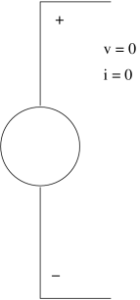Norator

In electronics, a norator is a theoretical linear time invariant one port which can have an arbitrary current and voltage between its terminals. A norator represents a controlled voltage or current source with infinite gain.

Inserting a norator in a circuit schematic provides whatever current and voltage the outside circuit demands. For example, the output of an ideal opamp behaves as a norator, producing nonzero output voltage and current that meet circuit requirements despite a zero input.

A norator is often paired with a nullator to form a nullor.

Two trivial cases are worth noting: A nullator in parallel with a norator is equivalent to a short (zero voltage any current) and a nullator in series with a norator is an open circuit (zero current, any voltage).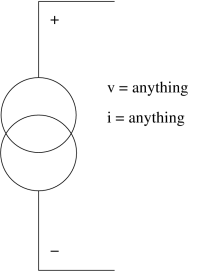Reference : Verhoeven C J M van Staveren A Monna G L E Kouwenhoven M H L & Yildiz E (2003)

## DC Circuit Analysis of a Capacitor

DC Circuit Analysis with a Capacitor :

In a DC circuit, if we open up a Capacitor, there will be no change in the circuit. We can analyse the circuit by this way.

If for example 9v passes through the Capacitor from a node, the exact 9v will cross the capacitor without any loss.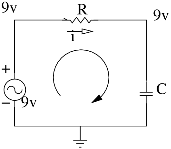An ideal capacitor is wholly characterized by a constant capacitance C, defined as the ratio of charge ±Q on each conductor to the voltage V between them.

Q=CV

Because the conductors (or plates) are close together, the opposite charges on the conductors attract one another due to their electric fields, allowing the capacitor to store more charge for a given voltage than if the conductors were separated, giving the capacitor a large capacitance.

Sometimes charge build-up affects the capacitor mechanically, causing its capacitance to vary. In this case, capacitance is defined in terms of incremental changes.

DC Condition :

In a network containing one or more capacitors, in a DC state it means that there are NO CURRENTS flowing through any branches in which a charged capacitor is located. Charged capacitors have voltage but not resistance: V= IR is not applicable since no currents flow THROUGH a capacitor. Voltages also correspond to zero.

dv/dt =0